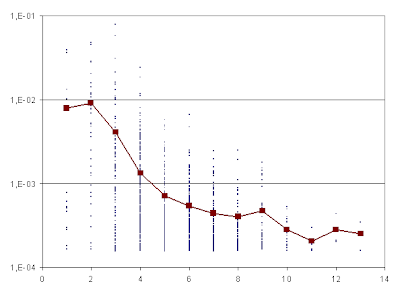## Friday, May 16, 2008

### Word frequency and length

What is the relationship between the length of a word and the frequency with which it appears in texts? At first thought it is apparent that longer words tend to be rarer, but we will try to estimate this relationship numerically.

A little Boost-based C++ program has been used to count the occurrences and length of the 1,000 most frequent words in two texts in different languages: Dickens' Our Mutual Friend and Leopoldo Alas' La Regenta. The results are shown in the figures above, where word frequency is plotted in logarithmic scale against word length.Fig. 1: Word frequency vs. word length, Our Mutual Friend.Fig. 2: Word frequency vs. word length, La Regenta.

The red line corresponds to the median frequency value as a function of word length: as expected, this frequency decreases as length grows, and seems to do so in an approximately exponential manner (or linear, if frequency is plotted on a logarithmic scale as above).

Another interesting way to look at this information is by estimating the entropy of the words with length n, in bits per character, for each value of n.The exact formula for this function is

f(n) := (1/n)∑wh(occurrences(w)/∑voccurrences(v)),

with w, v ranging over words of length n and h(x) defined as:

h(x) := −x·log2x.

The fact that the information per character diminishes as word length grows is explained by the relative sparsity of longer words, which is tantamount to saying that word spelling is more redundant as words grow longer.